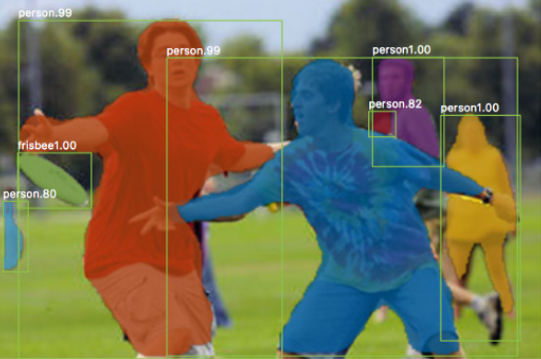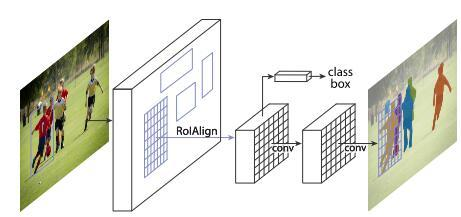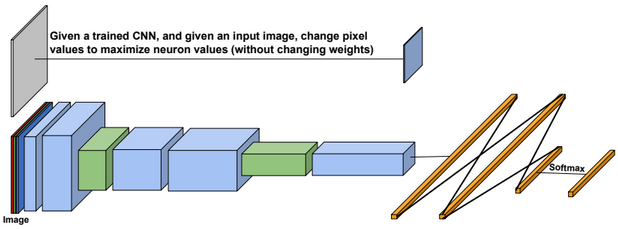# Computer Vision Datasets

Famous computer Vision datasets are: MNIST, ImageNet, CIFAR-10(0), Places.

Below the error rate for deep neural networks trained on the ImageNet dataset.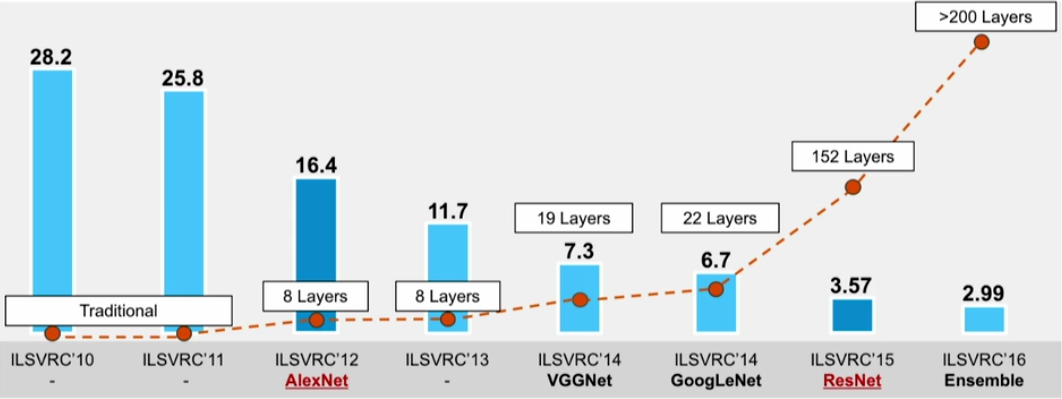# Convolution

A convolution is a neighborhood operation in which each output pixel is the weighted sum of neighboring input pixels. The matrix of weights is called the convolution kernel, also known as a filter.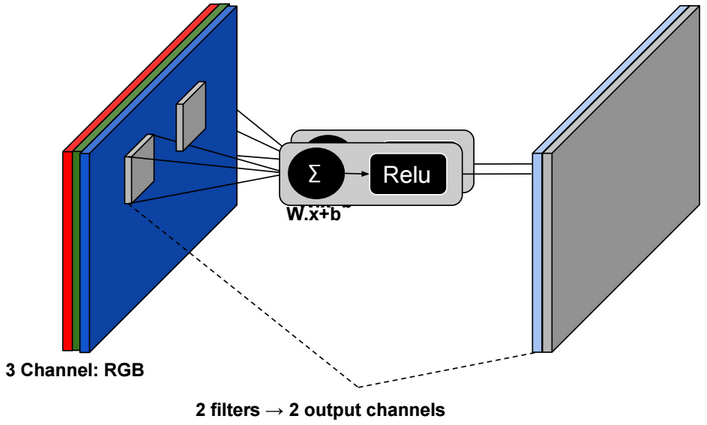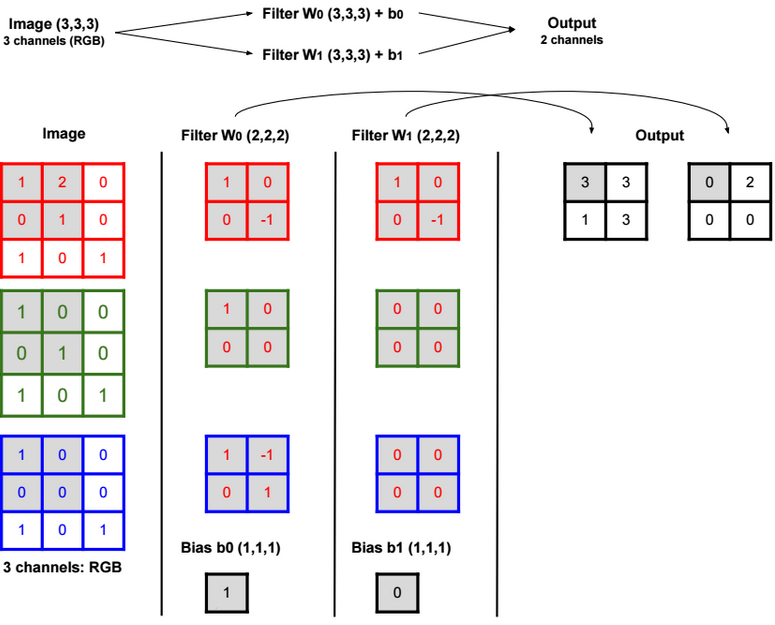Padding is basically adding rows or columns of zeros to the borders of an image input. It helps control the output size of the convolution layer. The formula to calculate the output size is: (N – F) / stride + 1.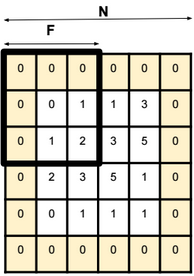For a 32x32x3 image and using 10 5×5 filters with stride 1 and pad 2, we get an output with size 32x32x10.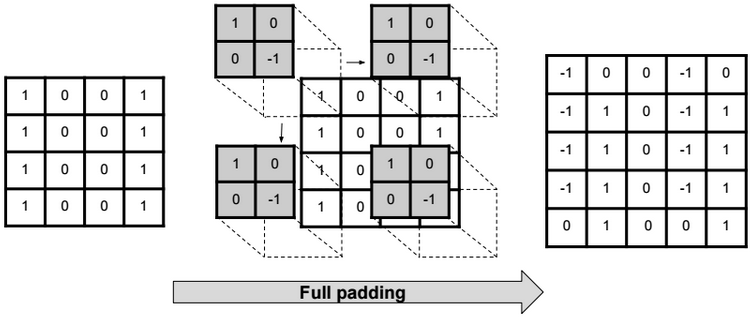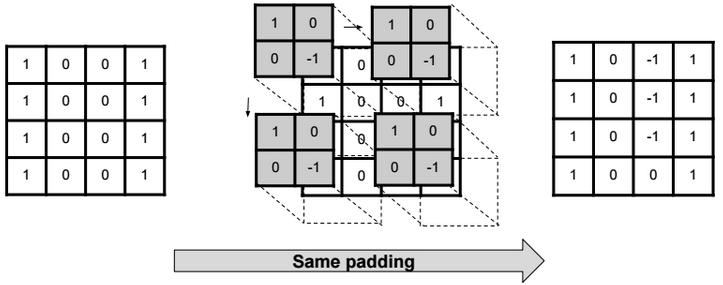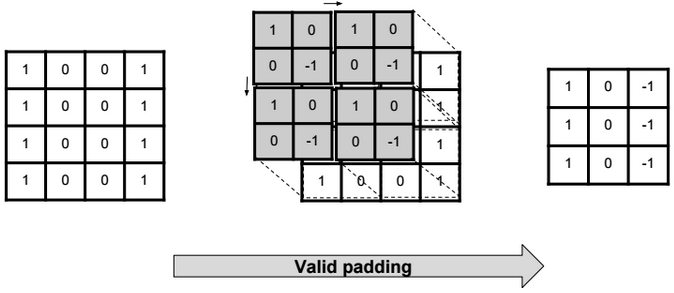# Transpose Convolution

Transpose Convolution (also called Deconvolution) is the reverse process of convolution.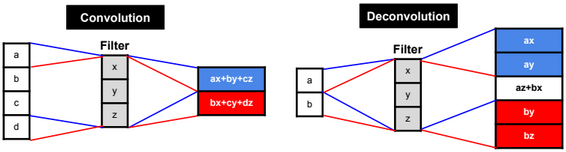The formula to calculate the output size is: stride.(input_w – 1) + ((input_w + 2.pad – kernel_w) mod stride) + kernel_w – 2.pad.

# Pooling

With pooling we reduce the size of the data without changing the depth.

Max pooling preserves edges.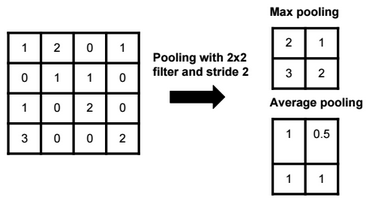The output size of a polling operation on a 8x8x10 representation using a 2×2 filter and with stride 2 is 4x4x10 (We can use the same formula: (N – F) / stride + 1).

Global Average pooling

Global Average pooling replaces all pixel values with one value per each channel. For example a Global Average Pooling of a 100×100 image with 3 channels (RGB) is a 1×1 image with 3 channels.

# Unpooling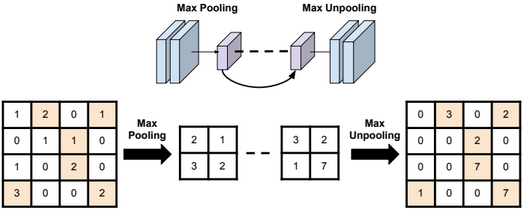Bed of nails unpooling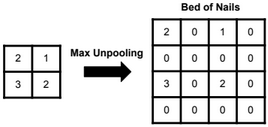Nearest neighbor unpooling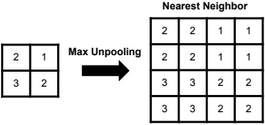# Architecture

In general the architecture of a convolution neural network is as below: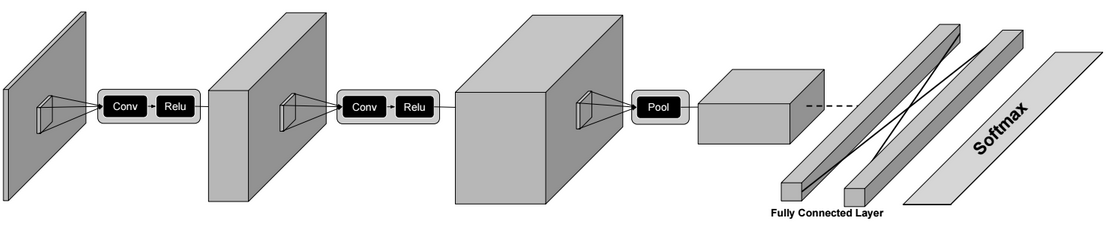Conv? Relu ? Conv ? Relu? Pool ? � ? Conv ? Fully Connected Layer ? Softmax

Some well known CNN architectures are: AlexNet (8 layers), VGG (16-19 layers), GoogLeNet (22 layers) and ResNet (152 layers).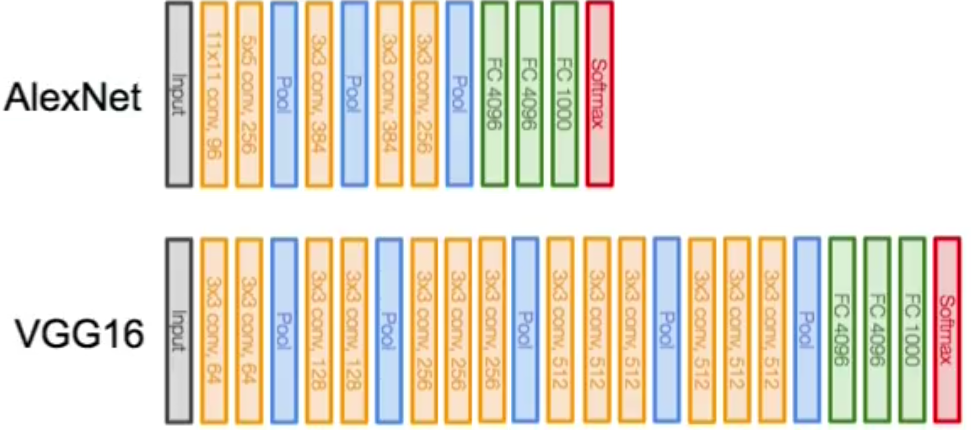For GoogleNet, the architecture is slightly different. It uses Inception modules which combine multiple parallel convolutions.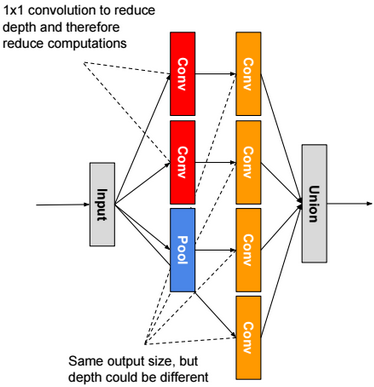For ResNet, we use residual blocks. The output of a residual block is the sum of the input X + the output of last convolution layer F(X). If weights are zeros, then the output of a residual block is the input X.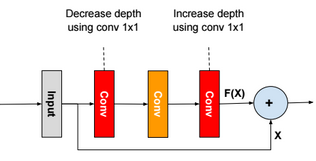There are other architectures like Network in Network, FractalNet, Densely Connected CNN, SqueezeNet.

Image Tagging

Basic classification of images.

Semantic Segmentation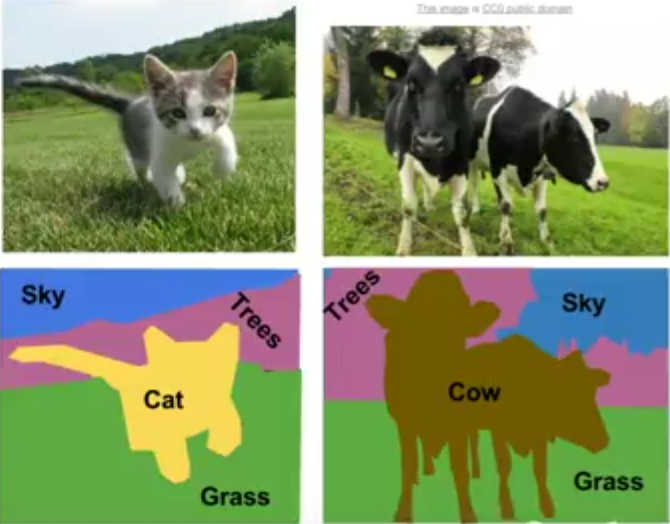Semantic segmentation is the task of assigning a class-label to each pixel in an image.

The general architecture of a CNN for this task is as follow: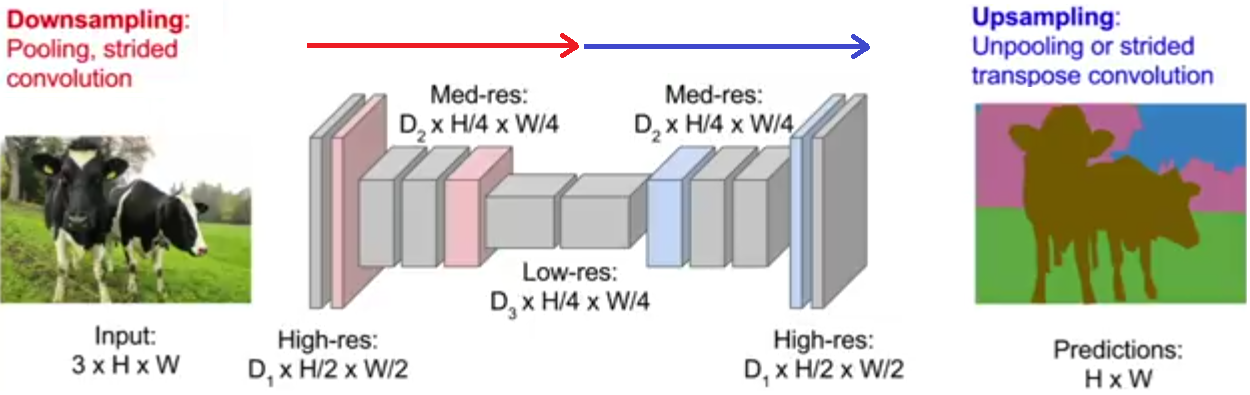In this task, we minimize the cross-entropy loss over every pixel.

Classification & Localisation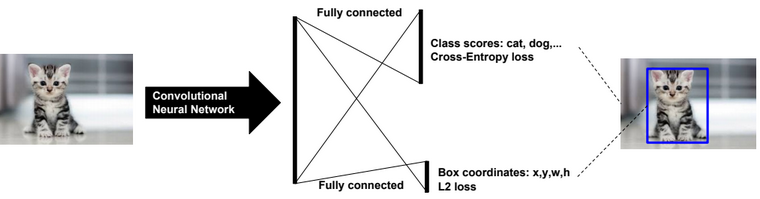Object Detection

Sliding window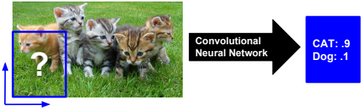Region proposals (selective search/R-CNN)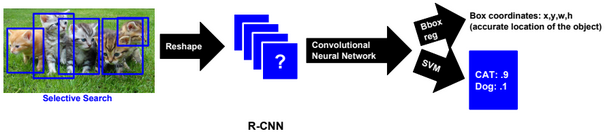Fast R-CNN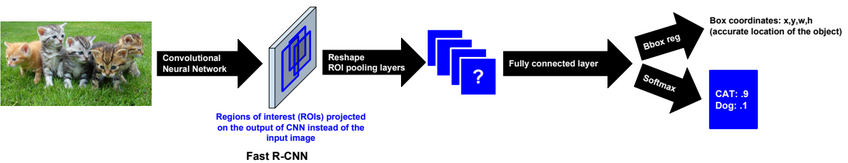Faster R-CNN

In Faster R-CNN we use and train a Region Proposal network instead of using selective search.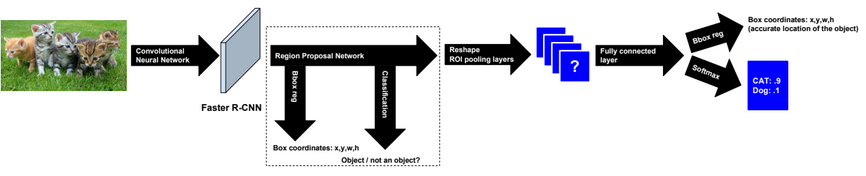Yolo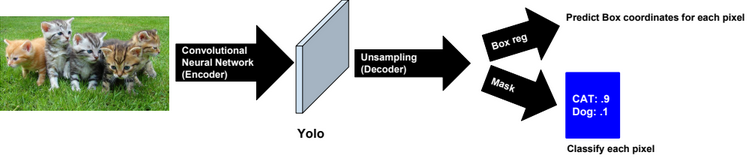It�s recommended to use Focal loss function when training the model.

Other methods

SSD

Instance Segmentation